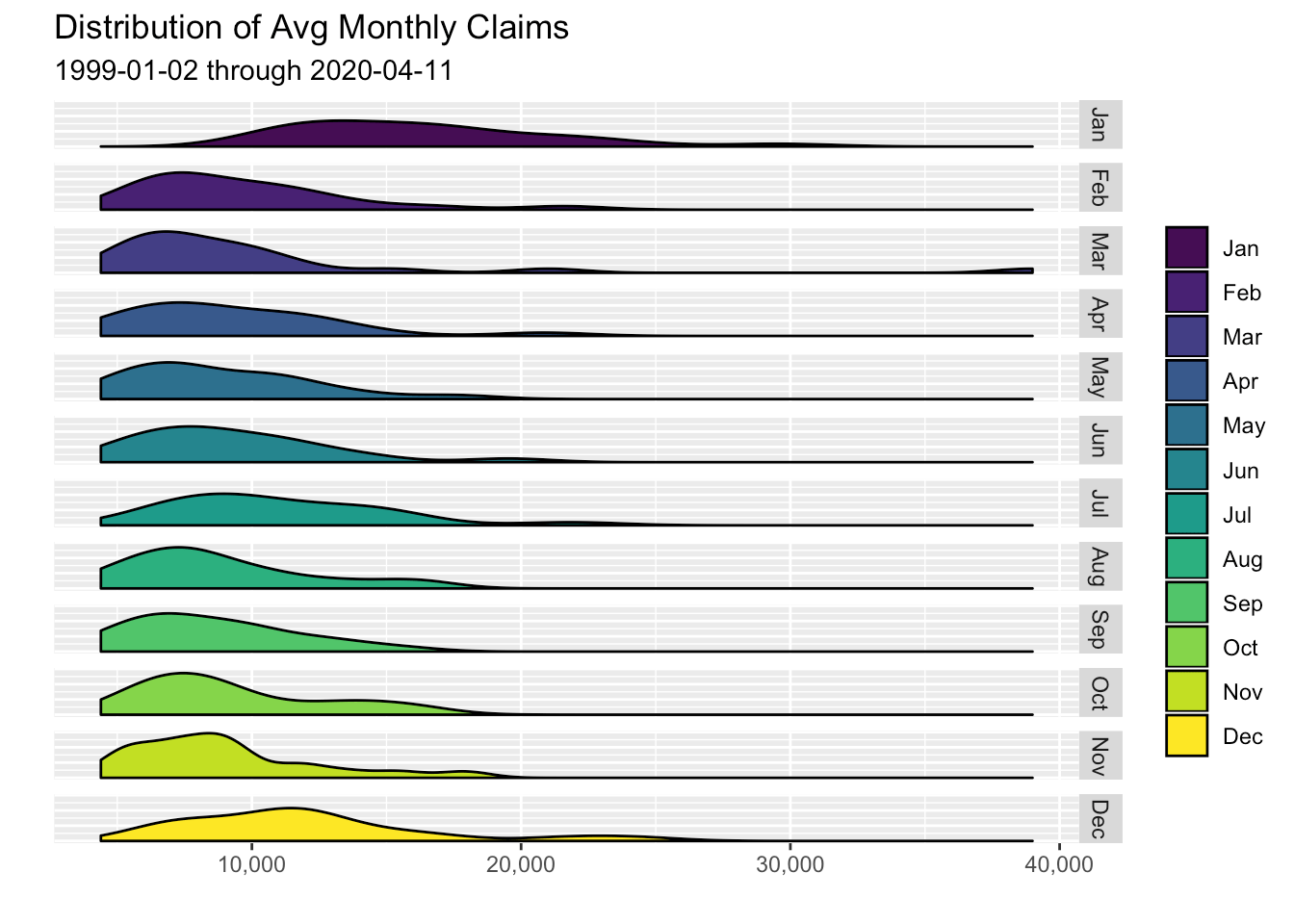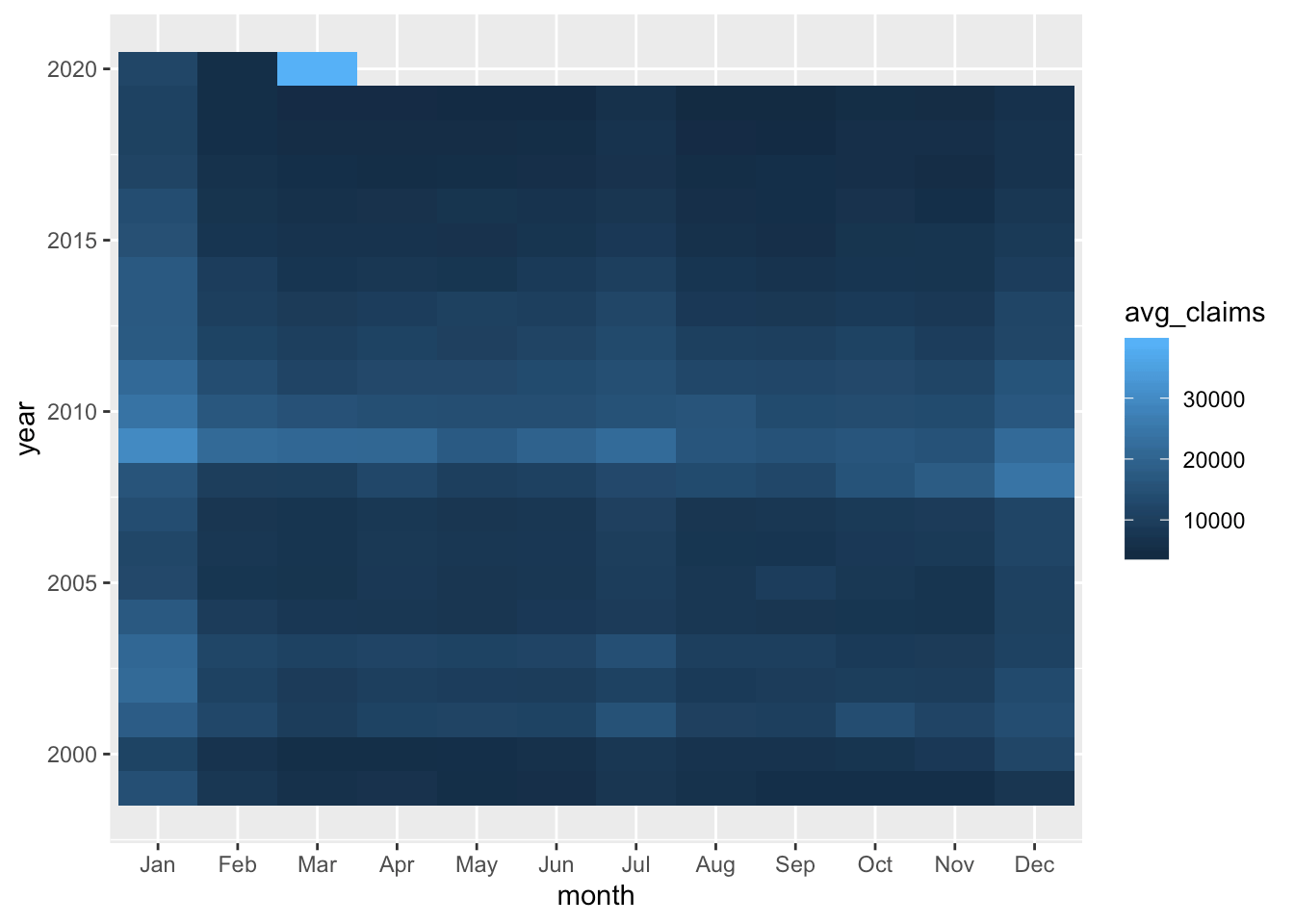Want to share your content on R-bloggers? click here if you have a blog, or here if you don't.

In today’s Reproducible Finance post, we will explore state-level unemployment claims which get released every Thursday. The last few weeks have shown huge spikes in those claims, of course, due to the coronavirus and statewide lockdown orders, and it got me wondering how these times will look to data scientists in the future.

Let’s start by importing unemployment insurance claims data for Georgia. This is a data series that’s reported by all 50 states.

We can grab this data from FRED using `ta_get()` from the `tidyquant` package. The FRED code for Georgia unemployment claims is `GAICLAIMS`.

``````ga_claims < -
"GAICLAIMS" %>%
tq_get(get = "economic.data",
from = "1999-01-01") %>%
rename(claims = price)

ga_claims %>%
slice(1, n())``````
``````# A tibble: 2 x 2
date       claims

1 1999-01-02   9674
2 2020-04-04 390132``````

For now, a quick visualization reveals what looks like a season pattern, with regular spikes in unemployment claims.

``````(
ga_claims %>%
ggplot(aes(x = date, y = claims)) +
geom_line(color = "cornflowerblue")  +
labs(
x = "",
y = "",
title = "Georgia Unemployment Claims",
subtitle = str_glue("{min(ga_claims\$date)} through {max(ga_claims\$date)}")
) +
theme_minimal() +
scale_y_continuous(labels = scales::comma)
) %>% ggplotly()``````

Let’s investigate this a bit further and look for a trend in average monthly claims by creating a series of faceted density plots. Notice how we can use `str_glue()` to pass in the dates for the subtitle, a nice trick learned from a Business Science Learning Lab that I use in almost all my plots now, either for titles, subtitles or hover text in `plotly`.

``````ga_claims %>%
mutate(
year = year(date),
month =  month(date, label = T, abbr  = T),
week = week(date)
) %>%
group_by(year, month) %>%
filter(n() >= 4) %>%
summarise(avg_claims = mean(claims)) %>%
ggplot(aes(x = avg_claims)) +
geom_density(aes(fill = as_factor(month))) +
facet_grid(rows = vars(as_factor(month))) +
guides(fill = guide_legend(title = "")) +
labs(
title = "Distribution of Avg Monthly Claims",
subtitle = str_glue("{min(ga_claims\$date)} through {max(ga_claims\$date)}"),
y = "",
x = ""
) +
theme(axis.text.y = element_blank(),
axis.ticks.y = element_blank()) +
scale_x_continuous(labels = scales::comma)``````January and December appear to have the density distribution most skewed to the right, meaning those months that have tended to show the highest number of unemployment claims.

Now let’s build a heat map to investigate months by year.

We’ll first create a column to hold the year and month for each observation.

``````ga_claims %>%
mutate(year = year(date),
month=  month(date, label = T, abbr  = T)) %>%
``````# A tibble: 6 x 4
date       claims  year month

1 1999-01-02   9674  1999 Jan
2 1999-01-09  19455  1999 Jan
3 1999-01-16  20506  1999 Jan
4 1999-01-23  12932  1999 Jan
5 1999-01-30  10871  1999 Jan
6 1999-02-06   7997  1999 Feb  ``````

Next, we calculate the average number of claims for each month of each year. We start with a `group_by(year, month)` before a call to `summarise()`.

``````ga_claims %>%
mutate(year = year(date),
month=  month(date, label = T, abbr  = T)) %>%
group_by(year, month) %>%
summarise(avg_claims = mean(claims)) %>%
``````# A tibble: 6 x 3
# Groups:   year 
year month avg_claims

1  1999 Jan       14688.
2  1999 Feb        7871.
3  1999 Mar        6095.
4  1999 Apr        6522.
5  1999 May        5451.
6  1999 Jun        5987.``````

That’s the data we want to chart but I want to add one more column with a slightly different format, one that uses a `k` for the thousands place, so we can stick these numbers into a chart. That is, instead of `14687.60`, I’d like to display `14.7K`.

We can use the `number_format()` function from the `scales` package to accomplish this. We set the `accuracy` to `.1` to indicate that we want to round off and show the `.1` decimal. We set `scale` to `1/1000` to indicate the scaling factor and choose `k` as the `suffix`.

``````ga_claims %>%
mutate(year = year(date),
month =  month(date, label = T, abbr  = T)) %>%
group_by(year, month) %>%
summarise(avg_claims = mean(claims)) %>%
mutate(
avg_claims_labels = scales::number_format(
accuracy = .1,
scale = 1 / 1000,
suffix = "k",
big.mark = ","
)(avg_claims)
) %>%
``````# A tibble: 6 x 4
# Groups:   year 
year month avg_claims avg_claims_labels

1  1999 Jan       14688. 14.7k
2  1999 Feb        7871. 7.9k
3  1999 Mar        6095. 6.1k
4  1999 Apr        6522. 6.5k
5  1999 May        5451. 5.5k
6  1999 Jun        5987. 6.0k             ``````

I called the new column `avg_claims_labels` because it’s a character column for display on the chart, not for any numerical use.

Here’s a first crack at the heat map. I’m going to put the months on the x-axis and years on the y-axis, and I want to `fill` according to `avg_claims`. Next we add a `geom_tile()`.

``````ga_claims %>%
mutate(year = year(date),
month =  month(date, label = T, abbr  = T)) %>%
group_by(year, month) %>%
filter(n() >= 4) %>%
summarise(avg_claims = mean(claims)) %>%
mutate(
avg_claims_labels = scales::number_format(
accuracy = 1,
scale = 1 / 1000,
suffix = "k",
big.mark = ","
)(avg_claims)
) %>%
ggplot(aes(
x = month,
y = year,
fill = avg_claims,
label = avg_claims_labels
)) +
geom_tile() ``````That gives us a sense that January has been the worst month in most years, and that 2009 was no picnic coming off the financial crisis. Let’s do a bit more cleanup by adding
`color = "white", size = .8, aes(height = 1)` to `geom_tile()`.

``````ga_claims %>%
mutate(year = year(date),
month =  month(date, label = T, abbr  = T)) %>%
group_by(year, month) %>%
filter(n() >= 4) %>%
summarise(avg_claims = mean(claims)) %>%
mutate(
avg_claims_labels = scales::number_format(
accuracy = 1,
scale = 1 / 1000,
suffix = "k",
big.mark = ","
)(avg_claims)
) %>%
ggplot(aes(
x = month,
y = year,
fill = avg_claims,
label = avg_claims_labels
)) +
geom_tile(color = "white", size = .8, aes(height = 1)) ``````We’re not done yet! I don’t love the shades of blue contrast here as it doesn’t really hammer home how bad a month March of 2020 was (Did your eye even get drawn to it? Mine didn’t initially), and we have not made use of our labels created with `number_format()`.

Let’s add our own fill colors with `scale_fill_gradient(low = "blue", high = "red", labels = scales::comma)`. If you’re wondering why I included `labels = scales::comma` when creating a gradient, it’s because I want the commas to show up in the legend.

We add our text labels with `geom_text()`, which picks up our `lable = avg_claims_labels` aesthetic. Let’s also add a `text()` aesthetic with `str_glue()` and pass the object to `ggplotly()` for interactivity.

``````(
ga_claims %>%
mutate(
year = year(date),
month =  month(date, label = T, abbr  = T)
) %>%
group_by(year, month) %>%
filter(n() >= 4) %>%
summarise(avg_claims = mean(claims)) %>%
mutate(
avg_claims_labels = scales::number_format(
accuracy = 1,
scale = 1 / 1000,
suffix = "k",
big.mark = ","
)(avg_claims)
) %>%
ggplot(
aes(
x = month,
y = year,
fill = avg_claims,
label = avg_claims_labels,
text = str_glue("average claims:
{scales::comma(avg_claims)}")
)
) +
geom_tile(color = "white", size = .8, aes(height = 1)) +
low = "blue",
high = "red",
labels = scales::comma
) +
geom_text(color = "white" , size = 3.5) +
theme_minimal() +
theme(
plot.caption = element_text(hjust = 0),
panel.grid.major.y = element_blank(),
legend.key.width = unit(1, "cm"),
panel.grid = element_blank()
) +
labs(
y  = "",
title = "Heatmap of Monthly Avg Unemployment Insurance Claims",
fill = "Avg Claims",
x = ""
) +
scale_y_continuous(breaks =  scales::pretty_breaks(n = 18))
) %>% ggplotly(tooltip = "text")``````

We can see that January is a terrible month, but March of 2020 is the worst month we’ve had in 20 years (though note I’m not adjusting for population growth in Georgia). We can use that hover text to embed whatever data we wish – for me, the magic of `str_glue()` has been a game changer.

We have done some work on monthly averages, but our recent experience might motivate us to dig in at the weekly level. For example, in each year since 1999, what has been the worst week of the year for unemployment claims? In the chart below, we’ll grab the worst week for each year, plotting it as a column whose height is equal to the number of claims, and coloring it by month.

``````(
ga_claims %>%
mutate(
month = month(date, label = TRUE, abbr = FALSE),
year = year(date)
) %>%
group_by(year) %>%
mutate(
max_claims = max(claims),
max_week_color = case_when(claims == max_claims ~ as.character(date),
TRUE ~ "NA")
) %>%
filter(max_week_color != "NA") %>%
ggplot(aes(
x = max_week_color,
y = claims,
fill = month,
text = str_glue("{date}
claims: {scales::comma(claims)}")
)) +
geom_col(width = .5) +
labs(
x = "",
title = str_glue("Highest Unemployment Claims Week, by Year
in Georgia"),
y = ""
) +
scale_y_continuous(
labels = scales::comma,
limits = c(0, NA),
breaks = scales::pretty_breaks(n = 6)
) +
scale_fill_brewer(palette = "Dark2") +
theme_minimal() +
theme(
axis.text.x = element_text(angle = 45),
plot.title = element_text(hjust = .5)
)
) %>% ggplotly(tooltip = "text")``````